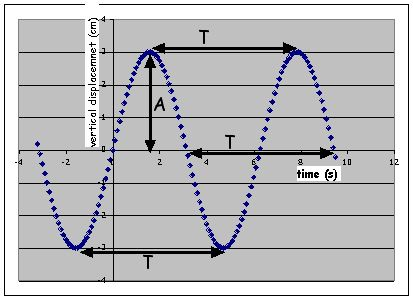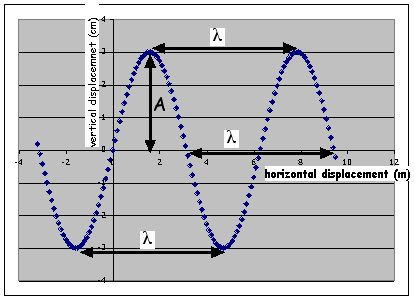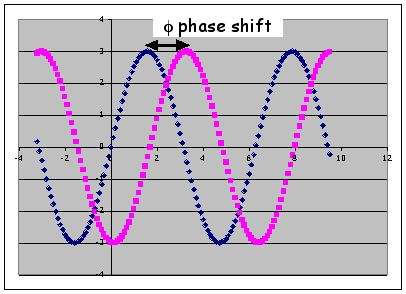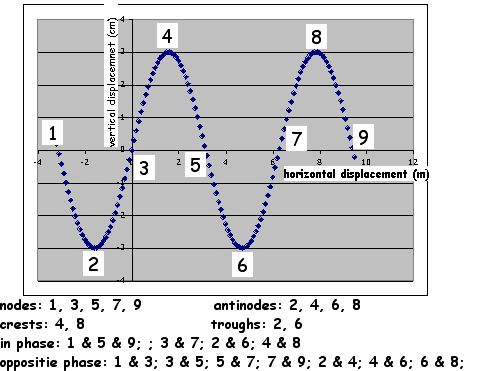## Waves

|SHM | Wave terms | Wave Phenomena | Extensions

associated with competency 10.2, 3, 4, and 5 for chap 13

The (mechanical) wave model: energy moves from one place to another with no net movement of particles. Energy will create a system that has frequency (f), period (T), wavelength (λ), velocity (v), amplitude (A), and angular frequency (ω). A pulse (a single disturbance that does not have frequency or wavelength) is used to illustrate many wave properities, but it is not a wave.

Mechanical waves require a source to create the wave, a medium to transmit the wave, and a receiver to detect the wave.

Christian Huygens (1629 - 1695) studied wave properties and produced several formal statements about waves. He was a contemporary of Sir Issac Newton and developed some competing ideas to explain observations involving light.

 mechanical waves: transverse, longitudinal, and torsional electromagnetic waves: (do not require a medium) only transverse waves mechanical waves: The source of the wave determines 1) the type of wave, 2) the frequency (f or ν) of the wave (which is also related to the period T), and 3) the energy of the wave The medium determines the velocity of the wave The source and the medium together determine the amplitude (A) and the wavelength (λ). E ∝ A electromagnetic waves: are different from mechanical waves because: E ∝ f no medium is required amplitude has no real meaning all waves travel at the same speed in a vacuum transverse waves: displacement of the medium is perpendicular to the direction of propogation longitudinal waves: displacement of the medium is parallel or antiparallel to the direction of propogation torsional waves: displacement of the medium is around the direction of propogation (be careful that there is no perpendicular motion because that would be a transverse wave) wave appletUniversal Wave Equation v = Δx/Δt = λ/T = fλ for electromagnetic waves c = fλ where c = 3.00x108m/s Huygens Principle: Every point on a wave front acts as the source of the ongoing wave. One implication of this is that frequency remains constant for a wave as it propogates through a medium and even into other media. Circular Waves are created by point sources. As the wave propogates outward the radius of curvature increases (the curvature decreases because C a 1/R). As the circular wave continues to propogate the amplitude (energy) of the wave decreases. This is caused by absorption and because the energy that was used to create the wave is being distributed over a larger and larger distance (the circumference of the circular wave). This creates a smaller energy density (energy spread along the circumference of the circle - J/m) which reduces the amplitude. A ray is an arrow that is drawn to represent the direction of propogation of a wave. It is drawn perpendicular to the wave front. A circular wave requires an infinity number of rays to represent the direction of propogation. Plane waves (require 1 ray) can be created 4 ways: 1) a line source (but remember that a line is an infinite number of colinear points that have the same frequency, amplitude, and phase; each point creates a circular wave; therefore a plane wave is the interference of an infinite number of colinear, in phase circular waves) 2) Remember that a plane wave has zero curvature, therefore its radius of curvature is infinity. So simply go any inifinite distance from a point source. 3) Reflection of a circular wave (created bya point source) that originates at the focus of a concave (converging) reflector. 4) Refraction of a circular wave (created bya point source) that originates at the focus of a double-convex (converging) refractor (lens)